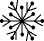EnchantedLearning.com is a user-supported site.
As a bonus, site members have access to a banner-ad-free version of the site, with print-friendly pages.

 You might also like: Divide Groups of Bugs Worksheet Printout Divide Twelve Farm Animals Worksheet Printout Divide Twelve Fall Items Worksheet Printout Snow Boot Joke: Add and Subtract, Then Decode the Riddle: Arithmetic Worksheet Math: Winter K-3 Theme Page Today's featured page: Pink

 Go to the AnswersMath Pages EnchantedLearning.com Divide Fifteen Icy Items Divide the icy items into groups to do division. Visual Division PagesDivision Pages

 Divide the snowmen into three equal groups.How many snowmen are there? How many groups of snowmen are there? How many snowmen are in each group?
15 ÷ 3 =

 Divide the igloos into five equal groups.How many igloos are there? How many groups of igloos are there? How many igloos are in each group?
15 ÷ 5 =

 Divide the snowflakes into fifteen equal groups.How many snowflakes are there? How many groups of snowflakes are there? How many snowflakes are in each group?
15 ÷ 15 =

## Enchanted Learning Search

 Search the Enchanted Learning website for: